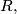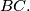### IMO Shortlist 1997 problem 18

Kvaliteta:
Avg: 0,0
Težina:
Avg: 6,0
The altitudes through the vertices$A,B,C$ of an acute-angled triangle$ABC$ meet the opposite sides at$D,E, F,$ respectively. The line through$D$ parallel to$EF$ meets the lines$AC$ and$AB$ at$Q$ and$R,$ respectively. The line$EF$ meets$BC$ at$P.$ Prove that the circumcircle of the triangle$PQR$ passes through the midpoint of$BC.$
Izvor: Međunarodna matematička olimpijada, shortlist 1997